Reducing the Dimensionality of Data with Neural Networks是对深度学习有重要影响的一篇论文，可以说是拉开了深度学习的帷幕，该论文出自 Hinton 大神之手。本文是读了论文后结合其他一些参考资料整理成的读书笔记。

## 自编码器与逐层预训练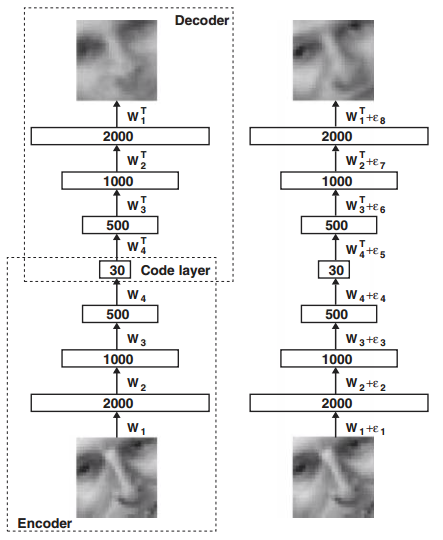### 逐层预训练过程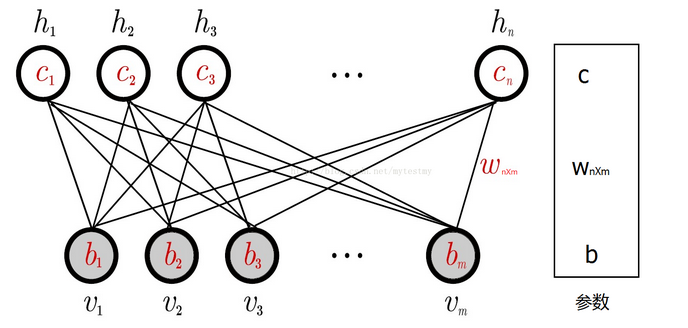RBM包含的参数有

• 权重矩阵 $W_{nm}$
• 隐藏层偏置量 $c = (c_1, c_2, c_3, … c_n)$
• 可视层偏置量 $b = (b_1, b_2, b_3, … b_m)$

$$\sigma(x) = 1/(1+e^{-x})\tag{3-3}$$

1. 正向过程：样本 $v$ 通过公式 (3-1) 从可视层输入得到 $h$
2. 反向过程：隐藏层 $h$ 通过公式 (3-2) 回传到可视层得到 $v’$, 利用 $v’$ 再进行一次正向传播得到隐藏层的 $h’$
3. 权重更新过程：更新公式为(其中 $\alpha$ 为学习率)
$$W(t+1) = W(t) + \alpha(vh^T-v’h’^T)\tag{3-4}$$
4. 迭代上面过程直至权重 $W$ 收敛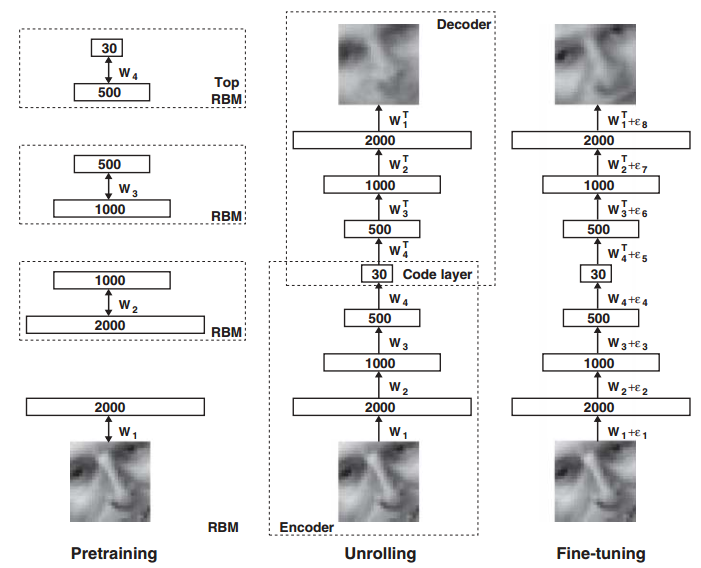### 原理与推导$$P(v,h) = \frac{e^{-E(v,h)}}{\sum_{v,h}e^{-E(v,h)}} \tag{3-6}$$

$$P(v) = \frac{\sum_he^{-E(v,h)}}{\sum_{v,h}e^{-E(v,h)}} \tag{3-7}$$
$$P(h) = \frac{\sum_ve^{-E(v,h)}}{\sum_{v,h}e^{-E(v,h)}} \tag{3-8}$$
$$P(v|h) = \frac{e^{-E(v,h)}}{\sum_ve^{-E(v,h)}} \tag{3-9}$$
$$P(h|v) = \frac{e^{-E(v,h)}}{\sum_he^{-E(v,h)}} \tag{3-10}$$

$$KL(q||p) = \sum_{x \epsilon \Omega} q(x) ln\frac{q(x)}{p(x)} =\sum_{x \epsilon \Omega} q(x)lnq(x)-\sum_{x \epsilon \Omega} q(x)lnp(x) \tag{3-11}$$

## 实验效果对比

### 实验一：曲线图像的压缩与恢复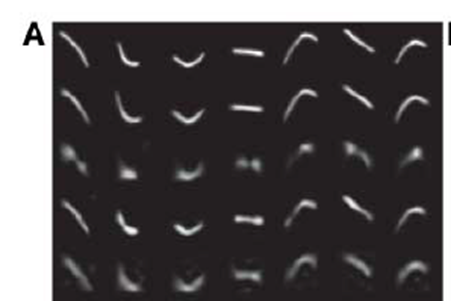PCA 6 7.64
Logistic PCA 18 2.45
PCA 18 5.90

### 实验二：手写数字图片的压缩、恢复与分类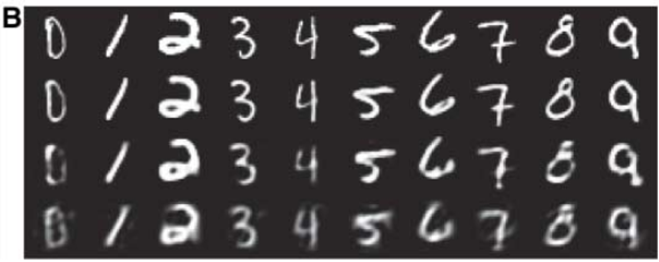Logistic PCA 30 8.01
PCA 30 13.87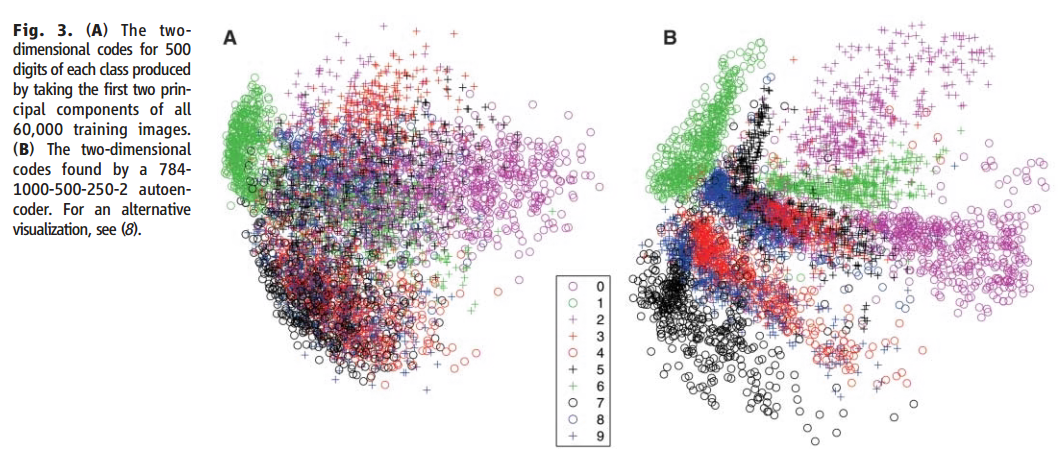### 实验三：人脸图像的压缩与恢复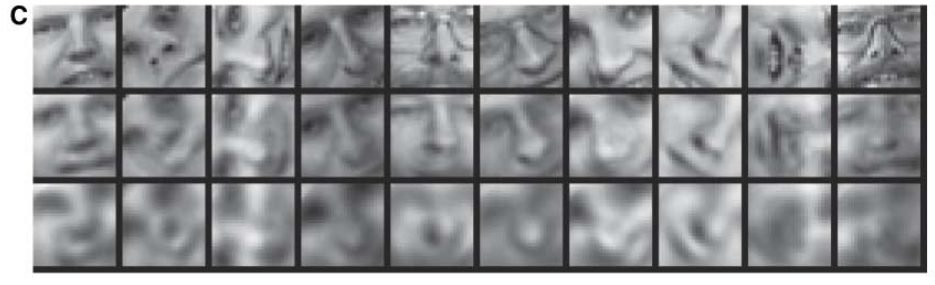PCA 30 135

### 实验四：词向量的降维与分类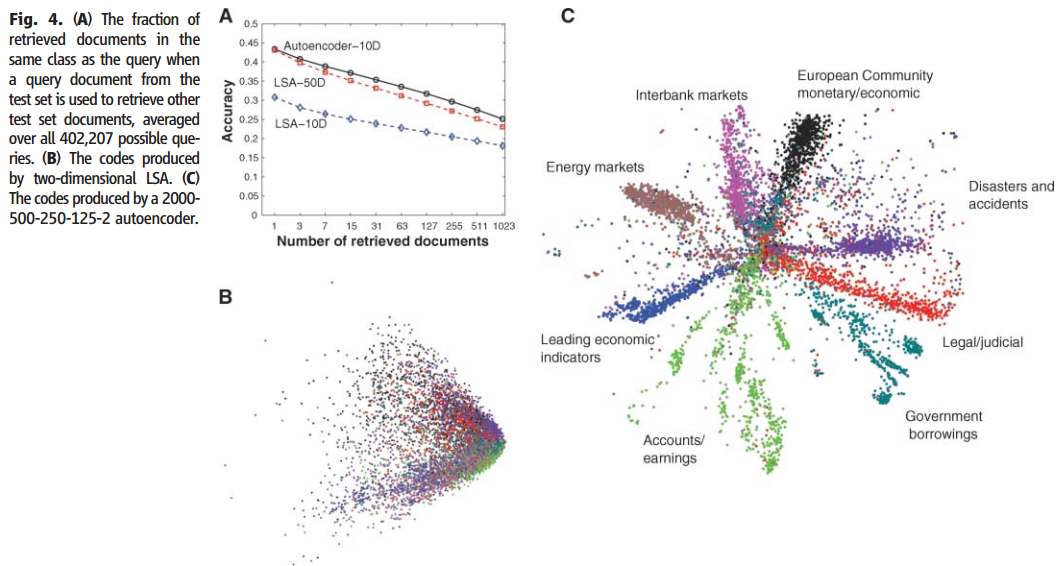## 总结

(1) 多层的神经网络具有优秀的特征学习能力，能够学习到数据更本质的特征
(2) 多层神经网络的初始化参数可通过逐层预训练获得